# CLASS 12 MATHS CHAPTER 11-THREE DIMENSIONAL GEOMETRY

### Solve The Following Questions.

Question1.If a line makes angles 90°, 135°, 45° with x ,y and z axes respectively, find its direction cosines.

Solution :

A-line makes 90°, 135°, 45°with x, y and z  axes respectively.

Therefore, Direction cosines of the line are cos 90°, cos135°, and cos45°

⇒ Direction cosines of the line are 0,-1/√2 and 1/√2

Question2.Find the direction cosines of a line which makes equal angles with the co-ordinate axes.

Solution :

Let the direction cosines of the line make an angle α with each of the coordinate axes.

∴ l = cos αm = cos αn = cos α

Thus, the direction cosines of the line, which is equally inclined to the coordinate axes, are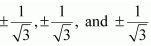Question3.If a line has direction ratios −18, 12, −4, then what are its direction cosines?

Solution :
The direction ratios of the lines are -18, 12, -4

Direction cosines of the lines are

Hence, direction cosine of line are -9/11, 6/11 -2/11.

Question4.Show that the points (2, 3, 4), (−1, −2, 1), (5, 8, 7) are collinear.

Solution :
The given points are A (2, 3, 4), B(−1, −2, 1) and C (5, 8, 7)

It is known that the direction ratios of line joining the points, (x1y1z1) and (x2y2z2), are given by, x2 − x1y2 − y1, and z2 − z1.

The direction ratios of AB are (−1 − 2), (−2 − 3), and (1 − 4) i.e., −3, −5, and −3.

The direction ratios of BC are (5 − (− 1)), (8 − (− 2)), and (7 − 1) i.e., 6, 10, and 6.

It can be seen that the direction ratios of BC are −2 times that of AB i.e., they are proportional.

Therefore, AB is parallel to BC. Since point B is common to both AB and BC, points A, B, and C are collinear.

Question5.Find the direction cosines of the sides of the triangle whose vertices are (3, 5, − 4), (− 1, 1, 2) and (− 5, − 5, − 2)

Solution :

The vertices of ΔABC are A (3, 5, −4), B (−1, 1, 2), and C (−5, −5, −2).

The direction ratios of side AB are (−1 − 3), (1 − 5), and (2 − (−4)) i.e., −4, −4, and 6.

Therefore, the direction cosines of AB are

The direction ratios of BC are (−5 − (−1)), (−5 − 1), and (−2 − 2) i.e., −4, −6, and −4.

Therefore, the direction cosines of BC are

The direction ratios of CA are 3−(−5), 5−(−5) and −4−(−2) i.e. 8, 10 and -2.

Therefore the direction cosines of CA are

### Solve The Following Questions.

Question 1.Show that the three lines with direction cosines  are mutually perpendicular.

Solution :

Two lines with direction cosines, l1m1n1 and l2m2n2, are perpendicular to each other, if l1l2 + m1m2 + n1n2 = 0

Therefore, the lines are perpendicular.

Thus, all the lines are mutually perpendicular.

Question 2.Show that the line through the points (1, −1, 2) (3, 4, −2) is perpendicular to the line through the points (0, 3, 2) and (3, 5, 6).

Solution :

Let AB be the line joining the points, (1, −1, 2) and (3, 4, − 2), and CD be the line joining the points, (0, 3, 2) and (3, 5, 6).

The direction ratios, a1b1c1, of AB are (3 − 1), (4 − (−1)), and (−2 − 2) i.e., 2, 5, and −4.

The direction ratios, a2b2c2, of CD are (3 − 0), (5 − 3), and (6 −2) i.e., 3, 2, and 4.

AB and CD will be perpendicular to each other, if a1a2 + b1b2c1c2 = 0

a1a2 + b1b2c1c2 = 2 × 3 + 5 × 2 + (− 4) × 4

= 6 + 10 − 16

= 0

Therefore, AB and CD are perpendicular to each other.

Question 3.Show that the line through points (4, 7, 8), (2, 3, 4) is parallel to the line through the points (−1, −2, 1), (1, 2, 5).

Solution :

Let AB be the line through the points, (4, 7, 8) and (2, 3, 4), and CD be the line through the points, (−1, −2, 1) and (1, 2, 5).

The directions ratios, a1b1c1, of AB are (2 − 4), (3 − 7), and (4 − 8) i.e., −2, −4, and −4.

The direction ratios, a2b2c2, of CD are (1 − (−1)), (2 − (−2)), and (5 − 1) i.e., 2, 4, and 4.

AB will be parallel to CD, if a1/a2 = b1/b2 = c1/c2

a1/a2 = -2/2 = -1

b1/b2 = -4/4 = -1

c1/c2 = -4/4 = -1

∴a1/a2 = b1/b2 = c1/c2

Thus, AB is parallel to CD.

Question 4.Find the equation of the line which passes through the point (1, 2, 3) and is parallel to the vector

Solution :

It is given that the line passes through the point A (1, 2, 3). Therefore, the position vector through A is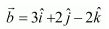It is known that the line which passes through point A and parallel to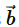is given by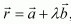λ is a constant.

This is the required equation of the line.

Question 5.Find the equation of the line in vector and in Cartesian form that passes through the point with position vector  and is in the direction

Solution :

It is given that the line passes through the point with position vector

It is known that a line through a point with position vector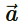and parallel tois given by the equation,This is the required equation of the line in vector form.

Eliminating λ, we obtain the Cartesian form equation as

Question 6.Find the Cartesian equation of the line which passes through the point (−2, 4, −5) and parallel to the line given by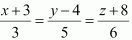Solution :

It is given that the line passes through the point (−2, 4, −5) and is parallel toThe direction ratios of the line, are 3, 5, and 6.

The required line is parallel to

Therefore, its direction ratios are 3k, 5k, and 6k, where k ≠ 0

It is known that the equation of the line through the point (x1y1z1) and with direction ratios, abc, is given byTherefore the equation of the required line is

Question7.The Cartesian equation of a line is  Write its vector form.

Solution :

The Cartesian equation of the line is

….(1)

The given line passes through the point (5, −4, 6). The position vector of this point is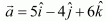Also, the direction ratios of the given line are 3, 7, and 2.

This means that the line is in the direction of vector,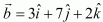It is known that the line through position vectorand in the direction of the vectoris given by the equation,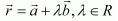This is the required equation of the given line in vector form.

Question 8.Find the vector and Cartesian equations of the line that passes through the origin and (5, −2, 3).

Solution :

The required line passes through the origin. Therefore, its position vector is given by,= 0                …..(1)

The direction ratios of the line through origin and (5, −2, 3) are

(5 − 0) = 5, (−2 − 0) = −2, (3 − 0) = 3

The line is parallel to the vector given by the equation,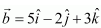The equation of the line in vector form through a point with position vectorand parallel tois,The equation of the line through the point (x1y1z1) and direction ratios abc is given by,Therefore, the equation of the required line in the Cartesian form is

Question 9.Find vector and Cartesian equations of the line that passes through the points (3, −2, −5), (3, −2, 6).

Solution :

Let the line passing through the points, P (3, −2, −5) and Q (3, −2, 6), be PQ.

Since PQ passes through P (3, −2, −5), its position vector is given by,

The direction ratios of PQ are given by,

(3 − 3) = 0, (−2 + 2) = 0, (6 + 5) = 11

The equation of the vector in the direction of PQ is

The equation of PQ in vector form is given by,The equation of PQ in Cartesian form isi.e.,

Question 10.Find the angle between the following pairs of lines:

(i)

(ii)

Solution :

(i) Let Q be the angle between the given lines.

The angle between the given pairs of lines is given by,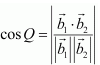The given lines are parallel to the vectors,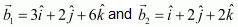, respectively.

(ii) The given lines are parallel to the vectors,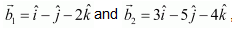respectively.

Question 11.Find the angle between the following pair of lines

(i)

(ii)

Solution :

Letand2 be the vectors parallel to the pair of lines, respectively.

(ii) Let12 be the vectors parallel to the given pair of lines,  , respectively.

Question 12.Find the values of p so that the lines   are at right angles.

Solution :
The given equations can be written in the standard form as

The direction ratios of the lines are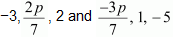respectively

Two lines with direction ratios, a1b1c1 and a2b2c2, are perpendicular to each other, if a1a2 + b1 b2 + c1c2 = 0

Question 13.Show that the lines  are perpendicular to each other.

Solution :

The equations of the given lines are

The direction ratios of the given lines are 7, −5, 1 and 1, 2, 3 respectively.

Two lines with direction ratios, a1b1c1 and a2b2c2, are perpendicular to each other, if a1a2 + b1 b2 + c1c2 = 0

∴ 7 × 1 + (−5) × 2 + 1 × 3

= 7 − 10 + 3

= 0

Therefore, the given lines are perpendicular to each other.

Question 14.Find the shortest distance between the lines

Solution :

The equations of the given lines are

Since, the shortest distance between the two skew lines is given by

Therefore, the shortest distance between the two lines is 3√2/2 units.

Question 15.Find the shortest distance between the lines  .

Solution :

The given lines are

It is known that the shortest distance between the two lines, is given by

Comparing this equation we have

Since distance is always non-negative, the distance between the given lines is 2√29 units.

Question 16.Find the shortest distance between the lines whose vector equations are

Solution :
The given lines are

It is known that the shortest distance between the lines,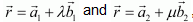is given by,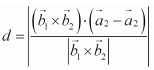…..(1)

Comparing the given equations with, we obtain

Substituting all the values in equation (1), we obtain

Therefore, the shortest distance between the two given lines is 3/√19 units.

Question 17.Find the shortest distance between the lines whose vector equations are

Solution :

The given lines are

It is known that the shortest distance between the lines,is given by,

For the given equations,

Substituting all the values in equation (3), we obtain

Therefore, the shortest distance between the lines is 8/√29 units.

### Solve The Following Questions.

Question1. In each of the following cases, determine the direction cosines of the normal to the plane and the distance from the origin.

(a) z = 2

(b) x + y + z = 1

(c) 2x + 3y – z = 5

(d) 5y + 8 = 0

Solution :
(The equation of the plane is z = 2 or 0x + 0y + z = 2 … (1)

The direction ratios of normal are 0, 0, and 1.

∴ √02 + 02 + 12 = 1

Dividing both sides of equation (1) by 1, we obtain

0.x + 0.y + 1.z = 2

This is of the form lx + my + nz = d, where lmn are the direction cosines of normal to the plane and is the distance of the perpendicular drawn from the origin.

Therefore, the direction cosines are 0, 0, and 1 and the distance of the plane from the origin is 2 units.

(b) x + y + z = 1 … (1)

The direction ratios of normal are 1, 1, and 1.

∴ √(1)² + (1)² + (1)² = √3

Dividing both sides of equation (1) by √3, we obtain

1/√3x + 1/√3y + 1/√3z = 1/√3               ….(2)

This equation is of the form lx + my + nz = d, where lmn are the direction cosines of normal to the plane and d is the distance of normal from the origin.

Therefore, the direction cosines of the normal are 1/√3,1/√3 and 1/√3the distance of normal from the origin is 1/√3 units.

(c) 2x + 3y ­− = 5 … (1)

The direction ratios of normal are 2, 3, and −1.

∴ √(2)² + (3)² + (-1)² = √14

Dividing both sides of equation (1) by √14 , we obtain

2/√14x + 3/√14y – 1/√14z = 5/√14

This equation is of the form lx + my + nz = d, where lmn are the direction cosines of normal to the plane and d is the distance of normal from the origin.

Therefore, the direction cosines of the normal to the plane are  2/√14x + 3/√14y – 1/√14z and the distance of normal from the origin is 5/√14  units.

(d) 5y + 8 = 0

⇒ 0x − 5y + 0z = 8 … (1)

The direction ratios of normal are 0, −5, and 0.

∴√0 +(-5)² + 0 = 5

Dividing both sides of equation (1) by 5, we obtain

-y = 8/5

This equation is of the form lx + my + nz = d, where lmn are the direction cosines of normal to the plane and d is the distance of normal from the origin.

Therefore, the direction cosines of the normal to the plane are 0, −1, and 0 and the distance of normal from the origin is 8/5 units.

Question 2. Find the vector equation of a plane which is at a distance of 7 units from the origin and normal to the vector

Solution :
The normal vector is,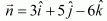It is known that the equation of the plane with position vector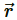is given by,= d

This is the vector equation of the required plane.

Question3. Find the Cartesian equation of the following planes:

(a)

(c)

Solution :
(a) It is given that equation of the plane is

For any arbitrary point P (xyz) on the plane, position vectoris given by,

Substituting the value ofin equation (1), we obtain

This is the Cartesian equation of the plane.

(b)

For any arbitrary point P (xyz) on the plane, position vectoris given by,

Substituting the value ofin equation (1), we obtain

This is the Cartesian equation of the plane.

(c)

For any arbitrary point P (xyz) on the plane, position vectoris given by,

Substituting the value ofin equation (1), we obtain

This is the Cartesian equation of the given plane.

Question4. In the following cases, find the coordinates of the foot of the perpendicular drawn from the origin:

(a) 2x + 3y + 4z – 12 = 0

(b) 3y + 4z – 6 = 0

(c) x + y + z = 1

(d) 5y + 8 = 0

Solution :

(a) Let the coordinates of the foot of perpendicular P from the origin to the plane be (x1y1z1).

2x + 3y + 4z − 12 = 0

⇒ 2x + 3y + 4z = 12 … (1)

The direction ratios of normal are 2, 3, and 4.

∴ √ 2² + 3² + 4² = √29

Dividing both sides of equation (1) by √29, we obtain

This equation is of the form lx + my + nz = d, where lmn are the direction cosines of normal to the plane and d is the distance of normal from the origin.

The coordinates of the foot of the perpendicular are given by

(ldmdnd).

Therefore, the coordinates of the foot of the perpendicular are

(b) Let the coordinates of the foot of perpendicular P from the origin to the plane be (x1y1z1).

3y + 4z – 6 = 0

⇒ 0x + 3y + 4y = 6 … (1)

The direction ratios of the normal are 0, 3, and 4.

∴ √0 + 3² + 4² = 5

Dividing both sides of equation (1) by 5, we obtain

0x + 3/5y + 4/5z = 6/5

This equation is of the form lx + my + nz = d, where lmn are the direction cosines of normal to the plane and d is the distance of normal from the origin.

The coordinates of the foot of the perpendicular are given by

(ldmdnd).

Therefore, the coordinates of the foot of the perpendicular are

(c) Let the coordinates of the foot of perpendicular P from the origin to the plane be (x1y1z1).

x + y + z = 1… (1)

The direction ratios of the normal are 1, 1, and 1.

√1² + 1² + 1² = √3

Dividing both sides of equation (1) by √3, we obtain

This equation is of the form lx + my + nz = d, where lmn are the direction cosines of normal to the plane and d is the distance of normal from the origin.

The coordinates of the foot of the perpendicular are given by

(ldmdnd).

Therefore, the coordinates of the foot of the perpendicular are

(d) Let the coordinates of the foot of perpendicular P from the origin to the plane be (x1y1z1).

5y + 8 = 0

⇒ 0x − 5y + 0z = 8 … (1)

The direction ratios of the normal are 0, −5, and 0.

√0 + (-5)2 + 0 = 5

Dividing both sides of equation (1) by 5, we obtain

-y = 8/5

This equation is of the form lx + my + nz = d, where lmn are the direction cosines of normal to the plane and d is the distance of normal from the origin.

The coordinates of the foot of the perpendicular are given by

(ldmdnd).

Therefore, the coordinates of the foot of the perpendicular are

Question5. Find the vector and Cartesian equations of the planes

(a) that passes through the point (1, 0, −2) and the normal to the plane is .

(b) that passes through the point (1, 4, 6) and the normal vector to the plane is .

Solution :

(a) The position vector of point (1, 0, −2) is

The normal vector N perpendicular to the plane is

The vector equation of the plane is given by,is the position vector of any point P (xyz) in the plane.

Therefore, equation (1) becomes

This is the Cartesian equation of the required plane.

(b) The position vector of the point (1, 4, 6) is

The normal vectorperpendicular to the plane is

The vector equation of the plane is given by,is the position vector of any point P (xyz) in the plane.

Therefore, equation (1) becomes

This is the Cartesian equation of the required plane.

Question 6. Find the equations of the planes that passes through three points:

(a) (1, 1, −1), (6, 4, −5), (−4, −2, 3)

(b) (1, 1, 0), (1, 2, 1), (−2, 2, −1)

Solution :
We know that through three collinear points A, B, C i.e., through a straight line, we can pass an infinite number of planes.

(a) The given points are A (1, 1, −1), B (6, 4, −5), and C (−4, −2, 3).

Since A, B, C are collinear points, there will be infinite number of planes passing through the given points.

(b) The given points are A (1, 1, 0), B (1, 2, 1), and C (−2, 2, −1).

Therefore, a plane will pass through the points A, B, and C.

It is known that the equation of the plane through the points,  is

This is the Cartesian equation of the required plane.

Question7. Find the intercepts cut off by the plane 2x + y – z = 5

Solution :

2x + y – z = 5                      ………(1)

Dividing both sides of equation (1) by 5, we obtain

It is known that the equation of a plane in intercept form is x/a + y/b + z/c = 1, where abc are the intercepts cut off by the plane at xy, and z axes respectively.

Therefore, for the given equation,

a = 5/2, b = 5 and c = -5

Thus, the intercepts cut off by the plane are 5/2, 5 and -5.

Question8. Find the equation of the plane with intercept 3 on the y- axis and parallel to ZOX plane.

Solution :

The equation of the plane ZOX is

y = 0

Any plane parallel to it is of the form, y = a

Since the y-intercept of the plane is 3,

∴ = 3

Thus, the equation of the required plane is y = 3

Question9. Find the equation of the plane through the intersection of the planes 3x – y + 2z – 4 = 0 and x + y + z – 2 = 0 and the point (2, 2, 1).

Solution :

The equation of any plane through the intersection of the planes,

3x − y + 2z ­− 4 = 0 and x + y + z − 2 = 0, is

The plane passes through the point (2, 2, 1). Therefore, this point will satisfy equation (1).

Question10. Find the vector equation of the plane passing through the intersection of the planes  and through the point (2, 1, 3).

Solution :

The equations of the planes are

The equation of any plane through the intersection of the planes given in equations (1) and (2) is given by,

The plane passes through the point (2, 1, 3). Therefore, its position vector is given by,

Substituting in equation (3), we obtain

This is the vector equation of the required plane.

Question11. Find the equation of the plane through the line of intersection of the planes x + y + z = 1 and 2x + 3y + 4z = 5 which is perpendicular to the plane x – y + z = 0

Solution :

The equation of the plane through the intersection of the planes, x + y + z = 1 and 2x + 3y + 4z = 5, is

(x + y + z – 1)  + λ(2x + 3y + 4z – 5)

⇒(2λ + 1)x + (3λ + 1)y + (4λ + 1)z – (5λ + 1) = 0                 …..(1)

The direction ratios, a1b1c1, of this plane are (2λ + 1), (3λ + 1), and (4λ + 1).

The plane in equation (1) is perpendicular to x – y + z = 0

Its direction ratios, a2b2c2, are 1, −1, and 1.

Since the planes are perpendicular,

Substituting λ = -1/3 in equation (1), we obtain

This is the required equation of the plane.

Question12. Find the angle between the planes whose vector equations are

Solution :

The equations of the given planes are

It is known that if n1 and n2 are normal to the planes,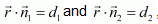then the angle between them, Q, is given by,

Question13. In the following cases, determine whether the given planes are parallel or perpendicular and in case they are neither, find the angle between them.

(a) 7x + 5y + 6z + 30 = 0 and 3x – y – 10z + 4 = 0

(b) 2x + y + 3z – 2 = 0 and x – 2y + 5 = 0

(c) 2x – 2y + 4z + 5 = 0 and 3x – 3y + 6z – 1

(d) 2x – y + 3z – 1 = 0 and 2x – y + 3z + 3 = 0

(e) 4x + 8y + z – 8 = 0 and y + z – 4 = 0

Solution :
The direction ratios of normal to the plane,, are a1b1c1 and .

(a) The equations of the planes are 7+ 5+ 6+ 30 = 0 and

3x − y − 10z + 4 = 0

Here, a1 = 7, b1 =5, c1 = 6

Therefore, the given planes are not parallel.

The angle between them is given by,

(b) The equations of the planes are 2x + y + 3z – 2 = 0 and x – 2y + 5 = 0

Thus, the given planes are perpendicular to each other.

(c) The equations of the given planes are 2x – 2y + 4z + 5 = 0 and 3x – 3y + 6z – 1

Here,

Thus, the given planes are not perpendicular to each other.

Thus, the given planes are parallel to each other.

(d) The equations of the planes are and 2x – y + 3z – 1 = 0 and 2x – y + 3z + 3 = 0

Thus, the given lines are parallel to each other.

(e) The equations of the given planes are4x + 8y + z – 8 = 0 and y + z – 4 = 0

Therefore, the given lines are not perpendicular to each other.

Therefore, the given lines are not parallel to each other.

The angle between the planes is given by,

Question14. In the following cases find the distances of each of the given points from the corresponding given plane:

(a) Point (0, 0, 0) Plane 3x – 4y + 12z = 3

(b) Point (3, −2, 1) Plane 2x – y + 2z + 3 = 0

(c) Point (2, 3, −5) Plane x + 2y – 2z = 9

(d) Point (−6, 0, 0) Plane 2x – 3y + 6z – 2 = 0

Solution :

It is known that the distance between a point, p(x1y1z1), and a plane, Ax + By + Cz = D, is given by,

(a) The given point is (0, 0, 0) and the plane is 3x – 4y + 12z = 3

(b) The given point is (3, − 2, 1) and the plane is 2x – y + 2z + 3 = 0

(c) The given point is (2, 3, −5) and the plane is x + 2y – 2z = 9

(d) The given point is (−6, 0, 0) and the plane is 2x – 3y + 6z – 2 = 0

### Solve The Following Questions.

Question 1.Show that the line joining the origin to the point (2, 1, 1) is perpendicular to the line determined by the points (3, 5, −1), (4, 3, −1).

Solution :

Let OA be the line joining the origin, O (0, 0, 0), and the point, A (2, 1, 1).

Also, let BC be the line joining the points, B (3, 5, −1) and C (4, 3, −1).

The direction ratios of OA are 2, 1, and 1 and of BC are (4 − 3) = 1, (3 − 5) = −2, and (−1 + 1) = 0

OA is perpendicular to BC, if a1a2 + b1b2 + c1c2 = 0

∴ a1a2 + b1b2 + c1c2 = 2 × 1 + 1 (−2) + 1 ×0 = 2 − 2 = 0

Thus, OA is perpendicular to BC.

Question2. If l1m1n1 and l2m2n2 are the direction cosines of two mutually perpendicular lines, show that the direction cosines of the line perpendicular to both of these are m1n2 − m2n1n1l2 − n2l1l1m2 ­− l2m1.

Solution :

It is given that l1m1n1 and l2m2n2 are the direction cosines of two mutually perpendicular lines. Therefore,

Let lmn be the direction cosines of the line which is perpendicular to the line with direction cosines l1m1n1 and l2m2n2.

lmn are the direction cosines of the line.

lm2 + n2 = 1 … (5)

It is known that,

Substituting the values from equations (5) and (6) in equation (4), we obtain

Thus, the direction cosines of the required line are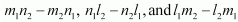Question3.Find the angle between the lines whose direction ratios are aband b − c, c − aa − b.

Solution :

The angle Q between the lines with direction cosines, abc and b − cc − a,

a − b, is given by,

Thus, the angle between the lines is 90°

Question 4.Find the equation of the line parallel to x-axis and passing through the origin.

Solution :

The line parallel to x-­­axis and passing through the origin is x-axis itself.

Let A be a point on x-axis. Therefore, the coordinates of A are given by (a, 0, 0), where a ∈ R.

Direction ratios of OA are (a − 0) = a, 0, 0

The equation of OA is given by,

Thus, the equation of line parallel to x-axis and passing through origin is

x/1 = y/0 = z/0

Question5.If the coordinates of the points A, B, C, D be (1, 2, 3), (4, 5, 7), (­−4, 3, −6) and (2, 9, 2) respectively, then find the angle between the lines AB and CD.

Solution :

The coordinates of A, B, C, and D are (1, 2, 3), (4, 5, 7), (­−4, 3, −6), and

(2, 9, 2) respectively.

The direction ratios of AB are (4 − 1) = 3, (5 − 2) = 3, and (7 − 3) = 4

The direction ratios of CD are (2 −(− 4)) = 6, (9 − 3) = 6, and (2 −(−6)) = 8

It can be seen that, a1/a2 = b1/b2 = c1/c2 = 1/2

Therefore, AB is parallel to CD.

Thus, the angle between AB and CD is either 0° or 180°.

Question6.If the lines  are perpendicular, find the value of k

Solution :

The direction of ratios of the lines, , are −3, 2k, 2 and 3k, 1, −5 respectively.

It is known that two lines with direction ratios, a1b1c1 and a2b2, c2, are perpendicular, if a1a2 + b1b2 + c1c2 = 0

Therefore, for k= -10/7, the given lines are perpendicular to each other.

Question7.Find the vector equation of the line passing through (1, 2, 3) and perpendicular to the plane

Solution :

The position vector of the point (1, 2, 3) is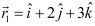The direction ratios of the normal to the plane, , are 1, 2, and −5 and the normal vector is

The equation of a line passing through a point and perpendicular to the given plane is given by,

Question8.Find the equation of the plane passing through (abc)  and parallel to the plane

Solution :

Any plane parallel to the plane, , is of the form            …….(1)

The plane passes through the point (abc). Therefore, the position vector  of this point is

Therefore, equation (1) becomes

Substituting in equation (1), we obtain

This is the vector equation of the required plane.

Substituting  in equation (2), we obtain

Question9.Find the shortest distance between lines

Solution :

The given lines are

It is known that the shortest distance between two lines,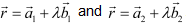is given by

Comparingto equations (1) and (2), we obtain

Substituting all the values in equation (1), we obtain

Therefore, the shortest distance between the two given lines is 9 units.

Question10.Find the coordinates of the point where the line through (5, 1, 6) and (3, 4, 1) crosses the YZ-plane.

Solution :

It is known that the equation of the line passing through the points, (x1y1z1) and (x2y2z2), is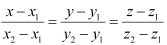The line passing through the points, (5, 1, 6) and (3, 4, 1), is given by,

Any point on the line is of the form (5 − 2k, 3k + 1, 6 −5k).

The equation of YZ-plane is x = 0

Since the line passes through YZ-plane,

5 − 2k = 0

Therefore, the required point is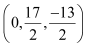.

#### Question11.Find the coordinates of the point where the line through (5, 1, 6) and (3, 4, 1) crosses the ZX-plane.

Solution :
It is known that the equation of the line passing through the points, (x1y1z1) and (x2y2z2), isThe line passing through the points, (5, 1, 6) and (3, 4, 1), is given by,

Any point on the line is of the form (5 − 2k, 3k + 1, 6 −5k).

Since the line passes through ZX-plane,

Question12. Find the coordinates of the point where the line through (3, ­−4, −5) and (2, − 3, 1) crosses the plane 2x + z = 7).

Solution :

It is known that the equation of the line through the points, (x1y1z1) and (x2y2z2), is

Since the line passes through the points, (3, −4, −5) and (2, −3, 1), its equation is given by,

Therefore, any point on the line is of the form (3 − kk − 4, 6k − 5).

This point lies on the plane, 2x + y + z = 7

∴ 2 (3 − k) + (k − 4) + (6k − 5) = 7

5k – 3 = 7

k = 2

Hence, the coordinates of the required point are (3 − 2, 2 − 4, 6 × 2 − 5) i.e.,

(1, −2, 7).

Question13. Find the equation of the plane passing through the point (−1, 3, 2) and perpendicular to each of the planes x + 2+ 3z = 5 and 3x + 3z = 0.

Solution :

The equation of the plane passing through the point (−1, 3, 2) is

(x + 1) + b (y − 3) + c (z − 2) = 0 … (1)

where, abc are the direction ratios of normal to the plane.

It is known that two planes,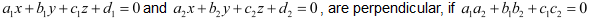Plane (1) is perpendicular to the plane, x + 2y + 3= 5

a.1 + b .2 + c.3 = 0

a + 2b + 3c = 0         ….(2)

Also, plane (1) is perpendicular to the plane, 3x + 3y + = 0

a .3 + b.3 + c.1 = 0

3a + 3b + c = 0          …..(3)

From equations (2) and (3), we obtain

Substituting the values of ab, and c in equation (1), we obtain

This is the required equation of the plane.

Question14. If the points (1, 1, p) and (−3, 0, 1) be equidistant from the plane   then find the value of p

Solution :

The position vector through the point (1, 1, p) is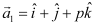Similarly, the position vector through the point (−3, 0, 1) is

The equation of the given plane is

It is known that the perpendicular distance between a point whose position vector isand the plane,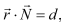is given by,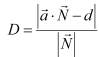Here,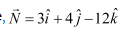and d = -13

Therefore, the distance between the point (1, 1, p) and the given plane is

Similarly, the distance between the point (−3, 0, 1) and the given plane is

It is given that the distance between the required plane and the points, (1, 1, p) and (−3, 0, 1), is equal.

∴ D1 = D2

Question15.Find the equation of the plane passing through the line of intersection of the planes   and  and parallel to x – axis.

Solution :
Equation of one plane is

The equation of any plane passing through the line of intersection of these planes is

Its direction ratios are (2λ + 1), (3λ + 1), and (1 − λ).

The required plane is parallel to x-axis. Therefore, its normal is perpendicular to x-axis.

The direction ratios of x-axis are 1, 0, and 0.

Substituting λ = -1/2 in equation (1), we obtain

Therefore, its Cartesian equation is y − 3z + 6 = 0

This is the equation of the required plane.

Question16. If O be the origin and the coordinates of P be (1, 2, −3), then find the equation of the plane passing through P and perpendicular to OP.

Solution :

The coordinates of the points, O and P, are (0, 0, 0) and (1, 2, −3) respectively.

Therefore, the direction ratios of OP are (1 − 0) = 1, (2 − 0) = 2, and (−3 − 0) = −3

It is known that the equation of the plane passing through the point (x1y1 z1) is

a(x – x1) + b(y – y1) + c(z – z1) = 0where, a, b, and c are the direction ratios of normal.

Here, the direction ratios of normal are 1, 2, and −3 and the point P is (1, 2, −3).

Thus, the equation of the required plane is

1(x – 1) + 2(y – 2) – 3(z + 3) 0

⇒ x + 2y – 3z – 14 = 0

Question17.Find the equation of the plane which contains the line of intersection of the planes   and which is perpendicular to the plane

Solution :

The equations of the given planes are

The equation of the plane passing through the line intersection of the plane given in equation (1) and equation (2) is

The plane in equation (3) is perpendicular to the plane,

Substituting λ = 7/19 in equation (3), we obtain

This is the vector equation of the required plane.

The Cartesian equation of this plane can be obtained by substituting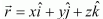in equation (3).

Question18.Find the distance of the point (−1, −5, −­10) from the point of intersection of the line  and the plane

Solution :

The equation of the given line is

…..(1)

The equation of the given plane is

…….(2)

Substituting the value of  from equation (1) in equation (2), we obtain

Substituting this value in equation (1), we obtain the equation of the line as

This means that the position vector of the point of intersection of the line and the plane is

This shows that the point of intersection of the given line and plane is given by the coordinates, (2, −1, 2). The point is (−1, −5, −10).

The distance d between the points, (2, −1, 2) and (−1, −5, −10), is

Question19.Find the vector equation of the line passing through (1, 2, 3)  and parallel to the plane  and

Solution :

Let the required line be parallel to vectorgiven by,

The position vector of the point (1, 2, 3) is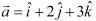The equation of line passing through (1, 2, 3) and parallel tois given by,

The equations of the given planes are

…..(2)

……(3)

The line in equation (1) and plane in equation (2) are parallel. Therefore, the normal to the plane of equation (2) and the given line are perpendicular.

From equations (4) and (5), we obtain

Therefore, the direction ratios ofare −3, 5, and 4.

Substituting the value ofin equation (1), we obtain

This is the equation of the required line.

Question20.Find the vector equation of the line passing through the point (1, 2, − 4) and perpendicular to the two lines:

Solution :

Let the required line be parallel to the vectorgiven by,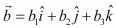The position vector of the point (1, 2, − 4) is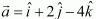The equation of the line passing through (1, 2, −4) and parallel to vectoris

The equations of the lines are

Line (1) and line (2) are perpendicular to each other.

Also, line (1) and line (3) are perpendicular to each other.

From equations (4) and (5), we obtain

Direction ratios ofare 2, 3, and 6.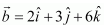Substitutingin equation (1), we obtain

This is the equation of the required line.

Question21.Prove that if a plane has the intercepts a,b,c and is at a distance of P units from the origin, then

Solution :

The equation of a plane having intercepts abc with xy, and z axes respectively is given by,

The distance (p) of the plane from the origin is given by,

Choose the correct answer in Exercise Q. 22 and 23.

Question22.Distance between the two planes: 2x + 3y + 4z = 4 and 4x + 6y + 8z = 12 is

(A) 2 units(B) 4 units(C) 8 units(D) 2/√29 units

Solution :

The equations of the planes are

2x + 3y + 4z = 4

4x + 6y + 8z = 12

⇒ 2x + 3y + 4z = 6

It can be seen that the given planes are parallel.

It is known that the distance between two parallel planes, ax by + cz = d1 and ax + by + cz = d2, is given by,

Thus, the distance between the lines is 2/√29 units.

Hence, the correct answer is D.

Question23. The planes: 2− y + 4z = 5 and 5x − 2.5y + 10z = 6 are

(A) Perpendicular (B) Parallel (C) intersect y-axis

(D) passes through  (0,0,5/4)

Solution :

The equations of the planes are

2− y + 4z = 5 … (1)

5x − 2.5y + 10z = 6 … (2)

It can be seen that,

Therefore, the given planes are parallel.

Hence, the correct answer is B.

Get 30% off your first purchase!

X
error: Content is protected !!
Scroll to Top